Relative and Absolute Reference in Excel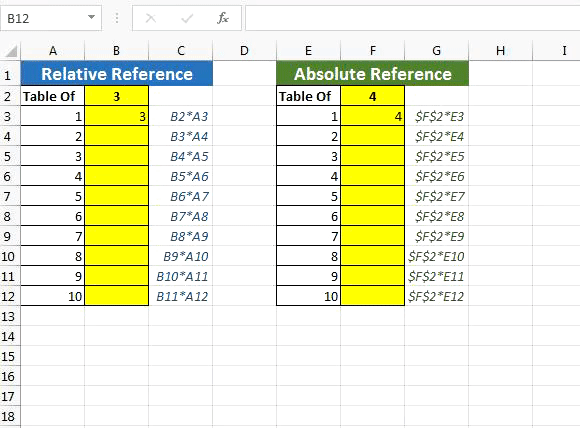While working or learning Excel, you’ve must heard of Relative and Absolute referencing of cells and ranges. Absolute referencing is also called locking of cells. Understanding of Relative and Absolute Reference in Excel is very important to work effectively on Excel. So lets get started.

Absolute Referencing or Locking Cell in Formula

When you don’t want to change the referenced cell while you copy a formula cell somewhere else, you use Absolute Referencing using \$ sign. Lets see an example.

Create A Table Generator

In this example you need to create a table generator. You will write a number in Cell B1. The Range B2: B11 should display the table of that number.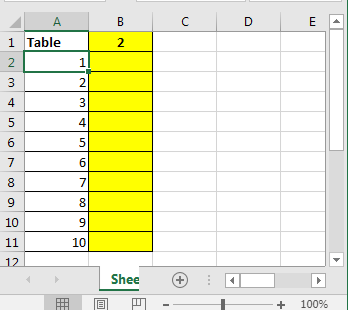You just need to multiply B1 to A1 and then just copy the formula below. It won’t work my friend but lets just try this…

In cell B2 write this formula and copy it in below cell.

=B1*A2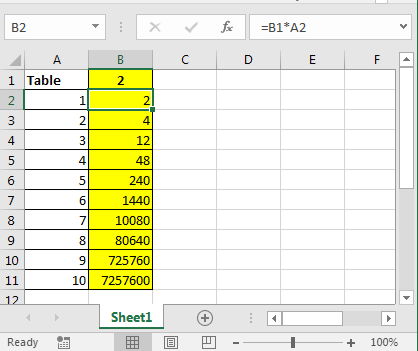What happened? ???? This is not the table of 2 you learned in primary school. Ok lets see whats going on here.

When copy or drag down the formula cell, the reference to B1 also changes. See below image.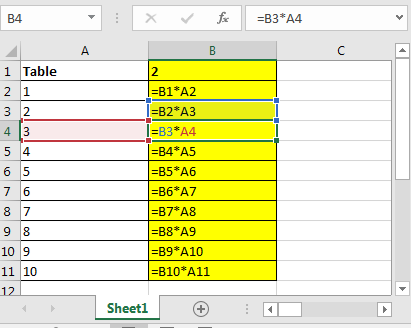This is called Relative Referencing. So how do we lock cell B2 or say give an Absolute Reference of a cell.

• Just put a \$ sign before column character and row number to lock the cell in excel. Eg. (\$A\$1).
• Or select the range in formula and hit F4 button.

The formula in cell B2 will look like this.

=\$B\$1*A2

Copy it in below cells or anywhere. The reference to B1 will not change.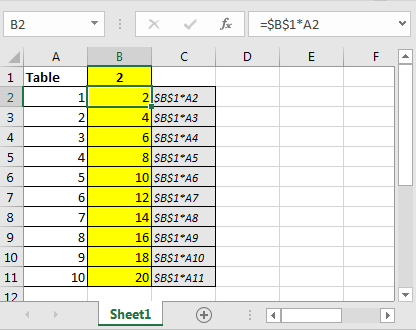Relative Referencing or Locking Cell in Formula

In above example, the reference to cell A2 is relative. The relative cell changes relatively when the formula is copied to another cell. As happens to A2 in the above example. Where the cell B2 is copied to B3 the reference of A2 changes to A3.

You can make row absolute and column relative or vice versa by just putting \$ sign before a column or row number.

For example eg; \$A1 means only column a is locked but row is not. If you copy this cell to left the column A will not change but when copy the formula downward the row number will change.

Terms and Conditions of use

The applications/code on this site are distributed as is and without warranties or liability. In no event shall the owner of the copyrights, or the authors of the applications/code be liable for any loss of profit, any problems or any damage resulting from the use or evaluation of the applications/code.